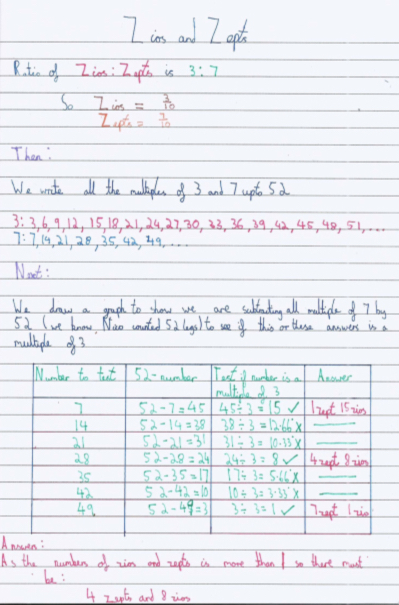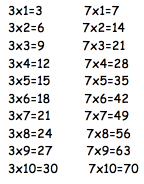#### You may also like### Chocolate

There are three tables in a room with blocks of chocolate on each. Where would be the best place for each child in the class to sit if they came in one at a time?### Four Triangles Puzzle

Cut four triangles from a square as shown in the picture. How many different shapes can you make by fitting the four triangles back together?### Cut it Out

Can you dissect an equilateral triangle into 6 smaller ones? What number of smaller equilateral triangles is it NOT possible to dissect a larger equilateral triangle into?

# Zios and Zepts

##### Age 7 to 11Challenge Level
We had a good number of solutions sent in for this challenge, here are a few to think about.

Jack from Whangaparaoa School in  New Zealand

3 x 1 = 3   52 - 3   = 49 divided by 7 = 7
3 x 8 = 24  52 - 24  = 28 divided by 7 = 4
3 x 15 = 45 52 - 45  = 7 divided by 7 = 1

Shivoham from Robin Hood Junior School sent in the following:From Hamilton South Public in Australia we had a few submitted solutions:

Henry:
Yes there are different ways to make 52, what I did was I had 7 Zepts and 1 Zios which makes 52 legs. But you can also make 52 legs with 4 x 7 Zepts = 28 legs and 3 x 8 = 24 so 28 + 24 = 52. So this shows there is more than 1 way to answer this question.

Caylum:
52 Legs would make 4 Zepts and 8 Zios

Finn:
In solving this problem of zepts and zois, there can be numerous answers to this problem. Some of the solutions include- 1 lot of zois and 7 lots of zeptsI worked this out by the  olution- 52/7= 7 with 3 left over  there for on of the solutions is 1 lot of zois and 7 lots of  Zepts. Another solution I came up with was- 15 lots of zois and 1 lot of zepts  I worked this solution out by swapping the numbers around from solution one- 52/3=15 with 7 left over there for another one of the solutions is 15 lots of zois and 1 lot of zepts. As you can see there are more than one answer I have demonstrated only one though.

Matilda.
I know my 3 times table found that 3 times 15 = 45 and 45 is 7 less that 52.
7 Times 7 is 49 which is 3 less than 52.

Jett :
I added up the legs by doing the Zepts legs and the Zios legs at the same time so
7+3=10 10+3+7=20 20+7=3=30 30+7+3=40 40+3+3+3+3=52
My other way was 7+7+7+7+7+7+7=49,  49+3=52
First answer 8 Zios 4 Zepts.
Second answer 1 Zios 7 Zepts. So there are 2 answers to the Zios and Zepts question.

Advika from Wimbledon Chase Primary School in England

There are 4 Zepts and 8 Zios
At first I tried to do it in ratio but it didn't work.I would write out the timetables of three and seven and then find out numbers that give a sum of fifty-two. Ratio does not work because (seven is to three )-the ratio- are not equal in one portion.My words may be rather. You could also approximate fifty two and use times tables to help you work
the main answer out. I could not find out any corresponding ways to get fifty two and would be grateful to get another answer that doesn't match mine. Below,I have uploaded a file that you may want to consider seeing as it should help you if you get stuck.I hope it is of help to you. Please consider my working out as I could not think of any other way to
come across the answer and would be happy to come across the method if
there is any. I hope this has come to use of you if any use.Looking at the tables above not many of them equal to 52 but I have found one that does. 24 (3x8=24)+28 (7x4=28)=52.
As a Ratio it can't work 7:3 is the ratio for Zepts to Zios. When you add them together it equals to 10 and 52 divided by 10 equals (5.2).Th is method does not work because each portion does not equal the same .This is because of the fact that Zepts equal to seven and Zios equals to three.

Amaan from Christ Church School England wrote:

7x4=28  4 Zepts - 3x8=24   8 Zios
I got this answer by trying to go up systematically in the 7 times table. First of I tried finding out how many Zios there were.Firstly,I took away 14 away from 52 because of the description in the clue. The answer to this sum was 38.From the answer of 38 I tried dividing 38 from 3.But there was no full answer. Next I tried 21,52-21=31. So once again my answer was not in the 3 times table. The next number in the 7 times table was 28. So once again took took my answer 28 away from 52.52-28=24.I then found out 8x3=24.So that was the answer to how many Zios there were and because 28 is the answer to 7x4 that told me the answer to how many Zepts there were.

Anaya from GEMS Willington Academy-AlKhail in the UAE:

As mentioned one zios has 3 legs and one zepts has 7 legs and  the scientist found total of 52 legs. We need to find the number of zios and zepts in the planet.
HERE IS THE SOLUTION
1 Zios    =   3 legs
8 zios  have   =  3 x 8 = 24 legs
1 zepts  = 7 legs
4 zepts have =  7x4=28 legs
So total  no of legs =24+28=52
So the answer is There are 8 NUMBER of zios and 4 NUMBER of zepts in the planet.

Great! You have worked hard on getting solutions and there are some good explanations as to how you went about finding solutions.